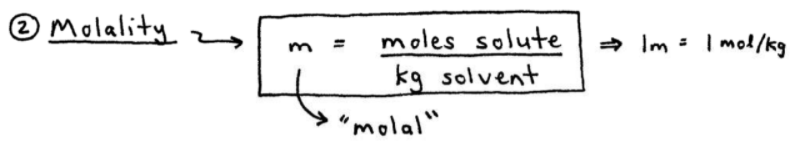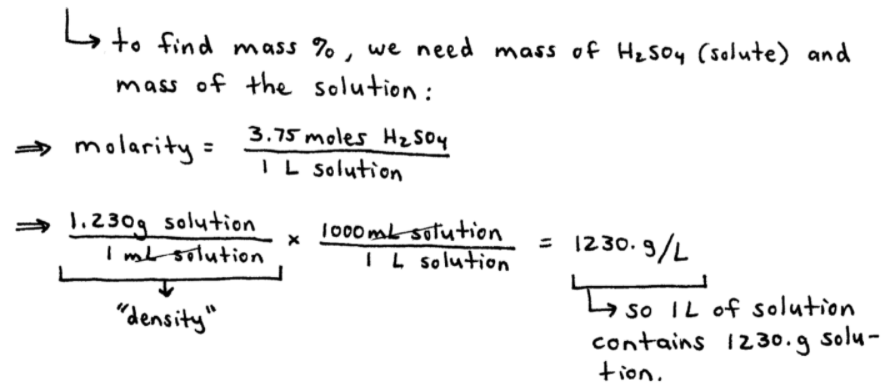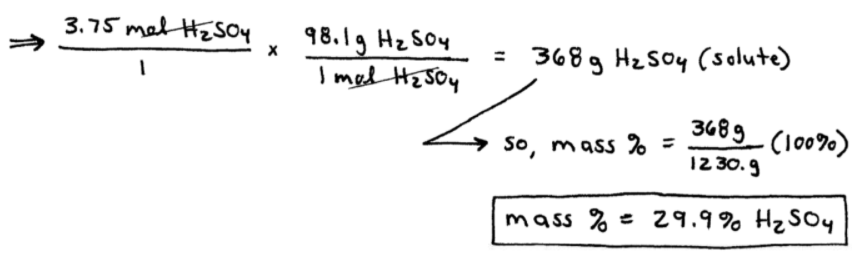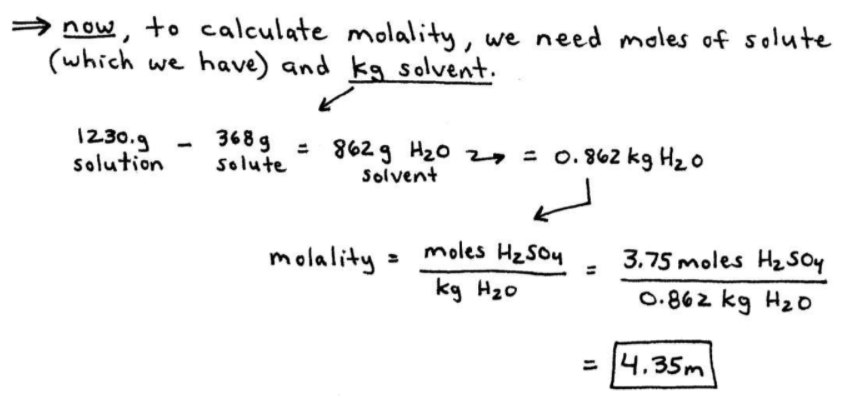# S11E1 - Types of Concentration. How to Calculate Molality and Mass Percent

Most substances we encounter are mixtures - wood, gas, milk, champagne, air, steel, etc...

When the components of a mixture are uniformly intermingled or mixed, the homogeneous mixture is a solution.

==========

## Solution Concentration

Solutions can be dilute or concentrated, but we need to define "solution composition" more precisely to do calculations.

There are several different ways to define a solution's concentration.

==========

## Types of Concentration

Here are five types of concentration...

• Molarity
• Molality
• Mass Percent
• Mole Fraction
• Normality

Let's take a closer look at each of these 5 types of concentration, and their respective formulas.

==========

## Molarity Formula

The molarity of a solution is equal to the moles of solute per one liter of solution.

Visually, we have the molarity formula below:Molarity is the most common way of determining (and providing) a solution's "composition" or concentration.

----------

## Molality Formula

The molality of a solution is equal to the moles of solute per one kilogram of solvent.

Here's how the molality formula looks:Most solutions in General Chemistry are dissolved in water, which means the solvent is H2O.

So molality often represents the amount of solute (in moles) per kilogram of water.

----------

## Mass Percent Formula

The mass percent formula of a solution is equal to the mass of the solute divided by the mass of the entire solution (both solute + solvent), times 100%.

Here's the formula for mass percent:This is just one chemistry teacher's opinion but, after molarity, mass percent is the second most common way to express the concentration of a solution in General Chemistry.

----------

## Mole Fraction Formula

The mole fraction of a solution is equal to the moles of solute divided by the moles of the entire solution (moles of solute + moles of solvent).

Visually, the mole fraction formula looks like this:Mole fraction is represented by an upper-case Greek letter "chi," which looks like a "wobbly X."

----------

## Normality Formula

The normality of a solution is equal to the number of equivalents of solute per one liter of solution.

The normality formula is here:Quite often, but not always, the normality of a solution is reserved for strong acid solutions and/or strong base solutions.

==========

## Mass Percent Calculations

Molarity calculation examples and mole fraction calculations can be found in my other videos.

But today, let's examine a mass percent calculation, followed by a typical molality calculation.

Here we go...

ex:  A sulfuric acid solution is 3.75 M and has a density of 1.230 g/mL.

Calculate the mass percent and molality of the sulfuric acid.
_________

➞ To find mass percent we need the mass of solute (H2SO4) and the mass of the solution

molarity = 3.75 moles H2SO4 / 1 L solution,

and...

(1.230 g solution / 1 mL solution) x (1000 mL / 1 L)

1230. g/L

➞ So, 1 L of solution contains 1230. g of solution.➞ Hold that for a second.

➞ Now, let's find the mass of solute, H2SO4, followed by the mass percent of H2SO4 .

We have:

(3.75 mol H2SO4 / 1) x (98.1 g H2SO4 / 1 mol H2SO4)

368 g H2SO4 (solute)

➞ Finally,

mass % =  [ (368 g H2SO4) / (1230. g solution) ] x 100%

29.9 % H2SO4

You can see it clearly below:----------

REMEMBER, we still have to do the second part of the problem: calculating the molality...

----------

## Molality Calculations

To calculate molality, we need moles of solute (which we have) and kg solvent.

1230. g solution  -  368 g solute  =  862 g H2O solvent

This is equal to = 0.862 kg H2O

➞ Molality  =  (moles H2SO4)  /  (1 kg H2O)

=  3.75 moles  /  0.862 kg

4.35 m H2SO4

Here's the above molality calculation, shown more visually:==========

Stick around for my next post from SECTION 11 - Solutions and Their Properties.

We'll discuss the